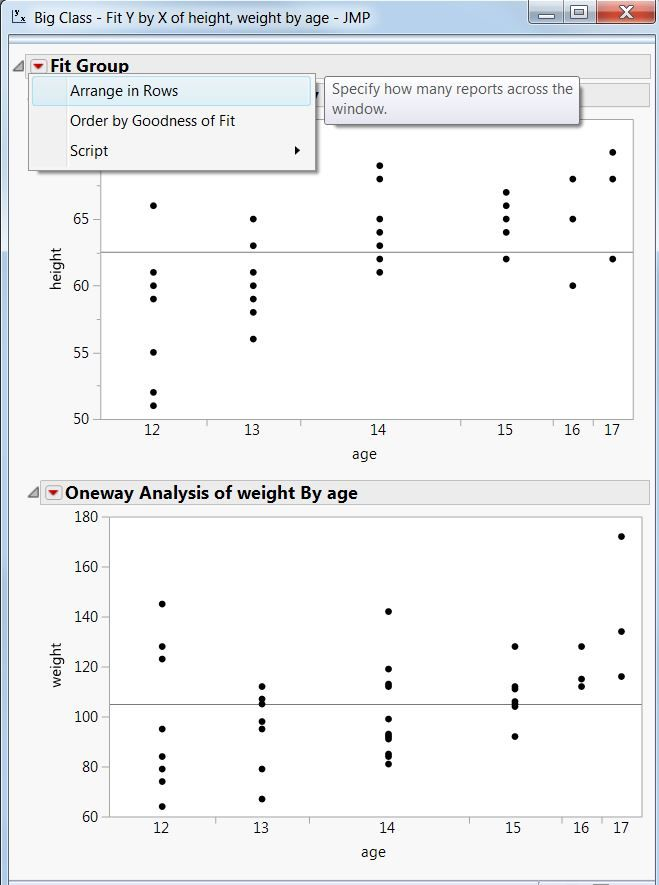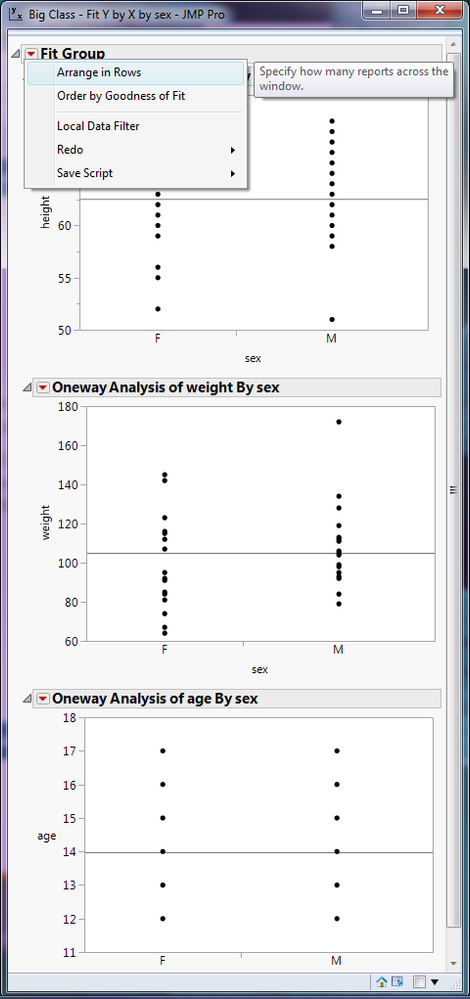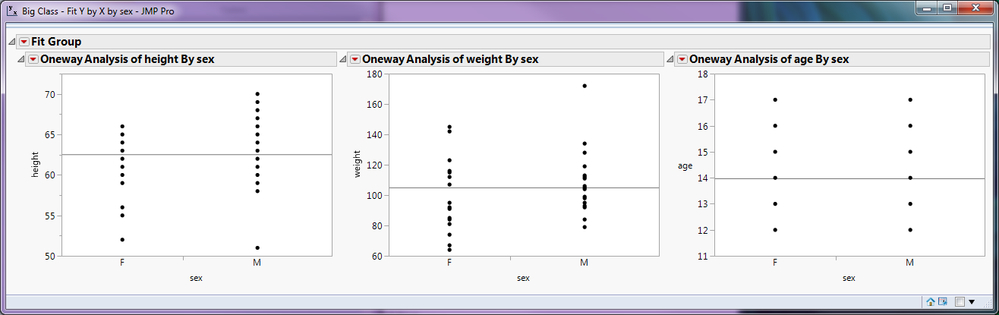The World Statistics Day celebration continues here in the Community. We all need reliable data for sound decision making. Do you have a data source that you trust most? Head over to Discussions to tell us about it.
Choose Language Hide Translation Bar
Highlighted

## Fit Y by X Graphs created in a row rather than column?

Hello,

The Fit Y by X when used to plot multiple Y,Responses and/or to plot multiple By variables, creates the plots in a single column.

I am wondering if there is way to make the plots appear in a row instead.

I have limited success trying to script such. But please if there are some methods to make this possible, please post.

thanks,

John

1 ACCEPTED SOLUTION

Accepted Solutions
Highlighted

## Re: Fit Y by X Graphs created in a row rather than column?

I am glad that the scripting option works for you, but regular JMP version 12 does still have the ability to arrange the graphs in rows. Perhaps this screen capture will show you where to click. The VERY TOP outline item will say Fit Group. That red pop-up will have the Arrange in Rows.Although this screen capture was on Oneway and not Bivariate, it looks exactly the same for Bivariate.

Dan Obermiller
14 REPLIES 14
Highlighted

## Re: Fit Y by X Graphs created in a row rather than column?

look under the red triangle, and select "Arange in Rows".

Jim
Highlighted

## Re: Fit Y by X Graphs created in a row rather than column?

Hi Jim,

in the Graph > Cell Plots menu I do find:  it seems X,Grouping warns when using continuous data, and prefers nominal or ordinal. (I am plotting continuous data.)

In Fit Y by X, in the plots I am making, there are four graphs produced and these graphs are in a single column aka one column and four rows in my output window. I have an interest to have the four graphs in a single row aka, one row and four columns.

Thanks,

John

Highlighted

## Re: Fit Y by X Graphs created in a row rather than column?

After you have run your plots in Fit Y by X, you can go to the red triangle in the output, and select Rarange in RowsWhich will give youWhat am I missing?

Jim
Highlighted

## Re: Fit Y by X Graphs created in a row rather than column?

Looks like what you're missing is you are using JMP Pro.

I am using JMP12. That is probably this issue.

At least that is what I suspect as to why I do not see that menu.

Highlighted

## Re: Fit Y by X Graphs created in a row rather than column?

I suspect you are correct.  Yes, I am running JMP Pro.  If running a script fits into your posibilities of a solution, here is a very simple script that will do what you want

``````Names Default To Here( 1 );
dt = Open( "\$SAMPLE_DATA/semiconductor capability.jmp" );

ycolList = {"NPN1", "PNP1", "NPN2", "PNP2"};

New Window( "FIT By Y Outputs",
H List Box(
For( I = 1, i <= N Items( Ycollist ), i++,
Oneway( Y( Column( dt,ycollist[i] ) ), X( :wafer ) )
)
)
)
;``````
Jim
Highlighted

## Re: Fit Y by X Graphs created in a row rather than column?

Hi Jim,

Example using the H List Box() in the following script does the trick:

////////
Names Default To Here( 1 );
dt = Open( "C:\Program Files\SAS\JMP\12\Samples\Data\Semiconductor Capability.jmp" );

dt:SITE << modeling type(continuous);
dt << select where (:lot_id == "lot1" | :lot_id == "lot2");
dt << invert row selection;
dt << hide;
dt << exclude;

dt << select where (:wafer == 1 | :wafer == 2);
dt << invert row selection;
dt << hide;
dt << exclude;
dt << clear select;

new window("example",
H List Box(
Bivariate( Y( :NPN1 ), X( :SITE ), by (:lot_id, :wafer) );
));

Highlighted

## Re: Fit Y by X Graphs created in a row rather than column?

Hi John,
You should see this function in JMP12 ("Arrange in Rows"). Click red triangle under "Fit Group".
Highlighted

## Re: Fit Y by X Graphs created in a row rather than column?

Could not find "Arrange in Rows" in the Bivariate Fit in JMP12. Maybe an example can show the detail.

However, the following script makes use the of horizontal list box:

////////
Names Default To Here( 1 );
dt = Open( "C:\Program Files\SAS\JMP\12\Samples\Data\Semiconductor Capability.jmp" );

dt:SITE << modeling type(continuous);
dt << select where (:lot_id == "lot1" | :lot_id == "lot2");
dt << invert row selection;
dt << hide;
dt << exclude;

dt << select where (:wafer == 1 | :wafer == 2);
dt << invert row selection;
dt << hide;
dt << exclude;
dt << clear select;

new window("example",
H List Box(
Bivariate( Y( :NPN1 ), X( :SITE ), by (:lot_id, :wafer) );
));

Highlighted

## Re: Fit Y by X Graphs created in a row rather than column?

Just as H List Box() created the horizontal layout that you prefer, Line Up Box( n cols, ... ) gives similar layout but allows you to have wrap the contents with n cols items per row.if it is getting too wide for you.

Learn it once, use it forever!
Article Labels

There are no labels assigned to this post.